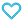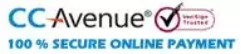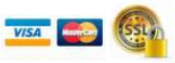# Higher Algebra-S.Barnard and J.M. Child

195.00

Concise theory supplemented with examples for better understanding of concepts. Use of lucid language makes it easy to understand. Chapter wise practise questions are given for better learning with answers.

57 in stock

Qty:Add to WishlistTable of Content :-
• 1. Theory of Numbers
• 2. Rationals and Irrationals
• 3. Polynomials
• 4. Symmetric and Alternating Functions, Substitutions
• 5. Complex Numbers
• 6. Theory of Equations
• 7. Partial Fractions
• 8. Summation of Series
• 9. Determinants
• 10. Systems of Equations
• 11. Reciprocal and Binomial Equations
• 12. Cubic and Biquadratic Equations
• 13. Theory of Irrationals
• 14. Inequalities
• 15. Sequences and Limits
• 16. Convergence of Series
• 17. Continuous Variable
• 18. Theory of Equations, Polynomials , Rational Fractions
• 19. Exponential and Logarithmic Functions and Series
• 20. Convergence
• 21. Binomial and Multinomial Theorems
• 22. Rational Fractions, Recurring Series and Difference Equations
• 23. The Operators , E, D. Interpolation
• 24. Continued Fractions
• 25. Indeterminate Equations of the First Degree
• 26. Theory of Numbers
• 27. Residues of Powers of a Number, Recurring Decimals
• 28. Numerical Solution of Equations
• 29. Implicit Functions, Curve Tracing
• 30. Infinite Products
• 31. Permutations, Combinations and Distributions
• 32. Probability
• 33. Continued Fractions
• MISCELLANEOUS EXERCISES (A), (B)